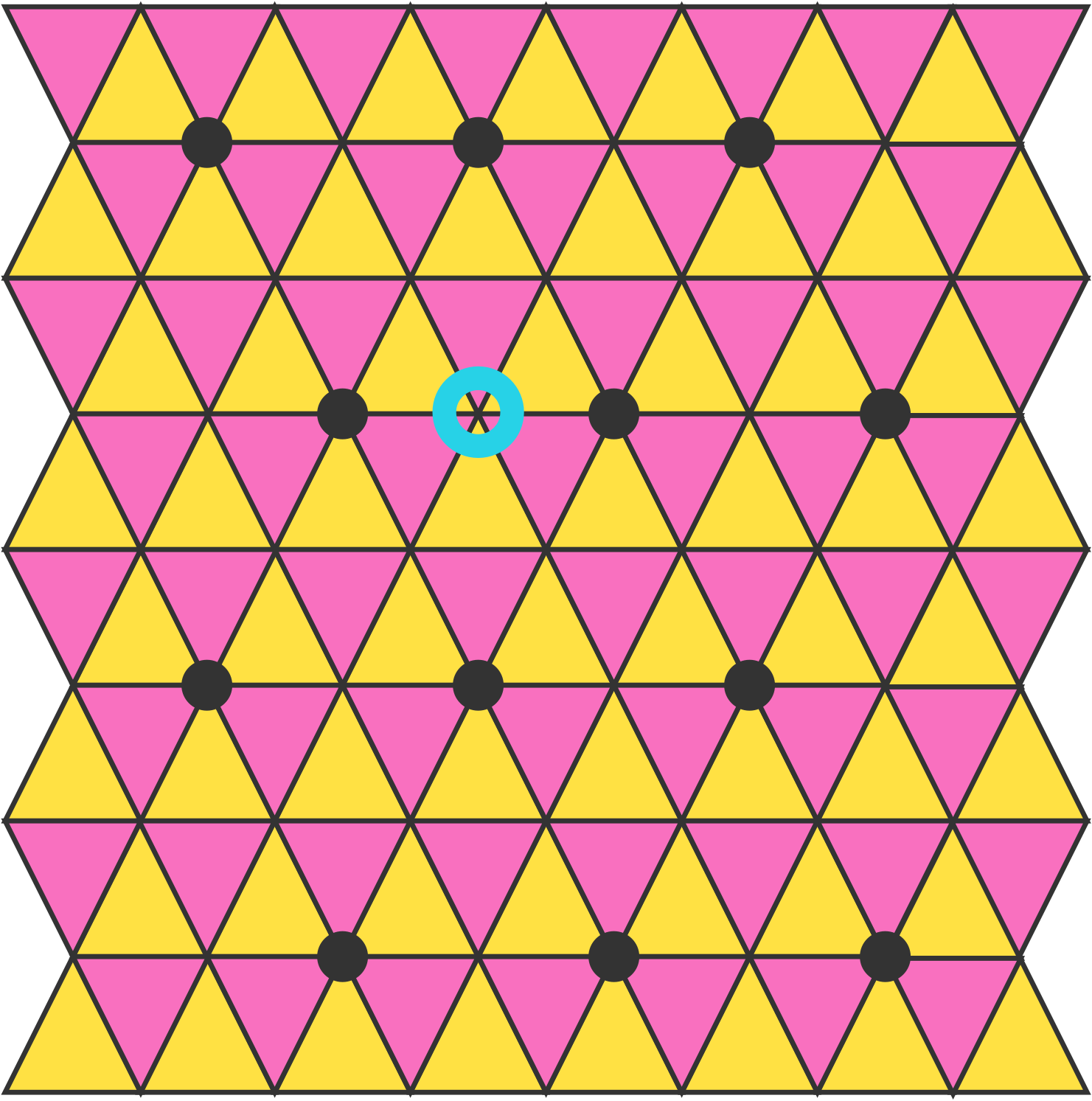Infinite Triangular Lattice

Probability Level 2

You are on an infinite triangular lattice with black circles repeated as shown below, and you start at the vertex circled in red:Every move you randomly walk along a black line segment to a neighboring vertex.

What is the expected value for the number of moves before you hit one of the lattice points with a black circle on it?

×